# RV'SMath

•### Descriptive Geometry - The Datum Line and The included Angles

Activity

RV'SMath

•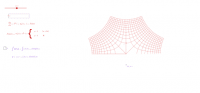### Single Term i Space

Activity

RV'SMath

•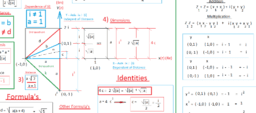### Complex Math

Book

RV'SMath

•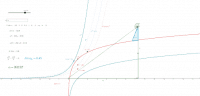### Dynamic Coordinates(i)

Activity

RV'SMath

•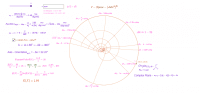### The Angle and its Points

Activity

RV'SMath

•### s(n+1)

Activity

RV'SMath

•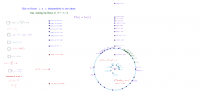### Plotting Points in the i - Space

Activity

RV'SMath

•### Hypotenuse of i

Activity

RV'SMath

•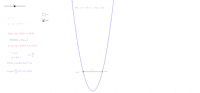### Plotting Parabols using Equations as Points

Activity

RV'SMath

•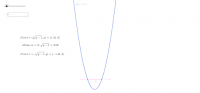### The points on x^2+1

Activity

RV'SMath

•### Points on the Parabola

Activity

RV'SMath

•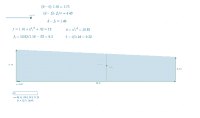### Version 2 - Area of a Trapezoid

Activity

RV'SMath

•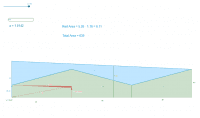### Version 1 - Area of Trapezoid

Activity

RV'SMath

•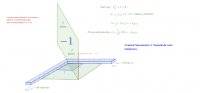### Graphical - Solving the Roots of x^2 + 1 = 0

Activity

RV'SMath

•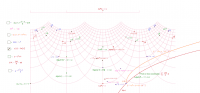### Points on the Complex Grid

Activity

RV'SMath

•### z^i

Activity

RV'SMath

•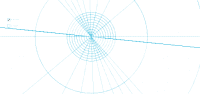### u^(i)v

Activity

RV'SMath

•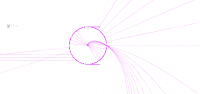### u^(iy)

Activity

RV'SMath

•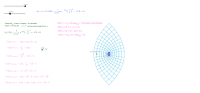### The Normal Curve in i - by the Numbers

Activity

RV'SMath

•### Dynamic Grids and their points

Activity

RV'SMath

•### Dynamic Grid

Activity

RV'SMath

•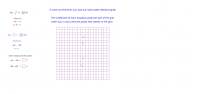### Plotting points on the grid in "y" and "x"

Activity

RV'SMath

•### Solving the roots of x^2 + 1 = 0

Activity

RV'SMath

•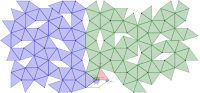Activity

RV'SMath